×

 方式： 出发城市： 到达城市： 出发日期： 到达日期： 单程 往返
 深圳 到 北京 往返未来1个月机票价格行情图 从 A 阿克苏 A 阿勒泰 A 安康 A 安庆 A 鞍山 A 安顺 A 安阳 B 保山 B 包头 B 北海 B 北京 B 北京南苑 B 蚌埠 C 长春 C 常德 C 昌都 C 长海 C 长沙 C 长治 C 常州 C 朝阳 C 承德 C 成都 C 赤峰 C 重庆 D 大理 D 大连 D 丹东 D 大同 D 达县 D 迪庆 D 东营 D 敦煌 E 恩施 F 富蕴 F 福州 G 赣州 G 格尔木 G 广汉 G 广元 G 广州 G 桂林 G 贵阳 H 海口 H 海拉尔 H 哈密 H 邯郸 H 杭州 H 汉中 H 哈尔滨 H 合肥 H 黑河 H 衡阳 H 和田 H 呼和浩特 H 黄山 H 黄岩 H 徽州 J 佳木斯 J 吉安 J 嘉峪关 J 吉林 J 济南 J 景德镇 J 井冈山 J 济宁 J 晋江 J 锦州 J 九江 J 酒泉 J 九寨沟 K 喀什 K 克拉玛依 K 库尔勒 K 昆明 K 昆山 K 库车 L 兰州 L 拉萨 L 梁平 L 连云港 L 丽江 L 临沧 L 林西 L 临沂 L 林芝 L 柳州 L 龙岩 L 洛阳 L 庐山 L 泸州 M 芒市 M 满洲里 M 梅县 M 绵阳 M 牡丹江 N 南昌 N 南充 N 南京 N 南宁 N 南通 N 南阳 N 宁波 P 攀枝花 Q 且末 Q 青岛 Q 秦皇岛 Q 庆阳 Q 齐齐哈尔 Q 泉州 Q 衢州 S 三亚 S 首尔 S 上海虹桥 S 上海浦东 S 鄯善 S 汕头 S 韶关 S 绍兴 S 沙市 S 沈阳 S 深圳 S 石家庄 S 石狮 S 思茅 S 苏州 T 塔城 T 太原 T 天津 T 通化 T 通辽 T 铜仁 U 乌兰浩特 U 乌鲁木齐 w 万现 W 潍坊 W 威海 W 温州 W 武汉 W 芜湖 W 无锡 W 武夷山 W 梧州 X 厦门 X 襄樊 X 西安咸阳 X 西昌 X 锡林浩特 X 兴城 X 兴宁 X 邢台 X 西宁 X 西双版纳 X 徐州 Y 延安 Y 盐城 Y 延吉 Y 烟台 Y 宜宾 Y 宜昌 Y 依兰 Y 银川 Y 伊宁 Y 义乌 Y 永州 Y 元谋 Y 榆林 Y 运城 Z 张家界 Z 湛江 Z 昭通 Z 郑州 Z 镇江 Z 芷江 Z 舟山 Z 珠海 Z 遵义 到 A 阿克苏 A 阿勒泰 A 安康 A 安庆 A 鞍山 A 安顺 A 安阳 B 保山 B 包头 B 北海 B 北京 B 北京南苑 B 蚌埠 C 长春 C 常德 C 昌都 C 长海 C 长沙 C 长治 C 常州 C 朝阳 C 承德 C 成都 C 赤峰 C 重庆 D 大理 D 大连 D 丹东 D 大同 D 达县 D 迪庆 D 东营 D 敦煌 E 恩施 F 富蕴 F 福州 G 赣州 G 格尔木 G 广汉 G 广元 G 广州 G 桂林 G 贵阳 H 海口 H 海拉尔 H 哈密 H 邯郸 H 杭州 H 汉中 H 哈尔滨 H 合肥 H 黑河 H 衡阳 H 和田 H 呼和浩特 H 黄山 H 黄岩 H 徽州 J 佳木斯 J 吉安 J 嘉峪关 J 吉林 J 济南 J 景德镇 J 井冈山 J 济宁 J 晋江 J 锦州 J 九江 J 酒泉 J 九寨沟 K 喀什 K 克拉玛依 K 库尔勒 K 昆明 K 昆山 K 库车 L 兰州 L 拉萨 L 梁平 L 连云港 L 丽江 L 临沧 L 林西 L 临沂 L 林芝 L 柳州 L 龙岩 L 洛阳 L 庐山 L 泸州 M 芒市 M 满洲里 M 梅县 M 绵阳 M 牡丹江 N 南昌 N 南充 N 南京 N 南宁 N 南通 N 南阳 N 宁波 P 攀枝花 Q 且末 Q 青岛 Q 秦皇岛 Q 庆阳 Q 齐齐哈尔 Q 泉州 Q 衢州 S 三亚 S 首尔 S 上海虹桥 S 上海浦东 S 鄯善 S 汕头 S 韶关 S 绍兴 S 沙市 S 沈阳 S 深圳 S 石家庄 S 石狮 S 思茅 S 苏州 T 塔城 T 太原 T 天津 T 通化 T 通辽 T 铜仁 U 乌兰浩特 U 乌鲁木齐 w 万现 W 潍坊 W 威海 W 温州 W 武汉 W 芜湖 W 无锡 W 武夷山 W 梧州 X 厦门 X 襄樊 X 西安咸阳 X 西昌 X 锡林浩特 X 兴城 X 兴宁 X 邢台 X 西宁 X 西双版纳 X 徐州 Y 延安 Y 盐城 Y 延吉 Y 烟台 Y 宜宾 Y 宜昌 Y 依兰 Y 银川 Y 伊宁 Y 义乌 Y 永州 Y 元谋 Y 榆林 Y 运城 Z 张家界 Z 湛江 Z 昭通 Z 郑州 Z 镇江 Z 芷江 Z 舟山 Z 珠海 Z 遵义

在线支付100%认证

全球机票联网售票

• ## 全天服务

7*24小时专业客服人员为您服务

• ## 方便快捷

电子客票，身份证直接换登机牌

http://www.yuexingjp.com | 全国免费统一服务热线400-1688935 | 上海地区 |在线咨询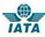经营性网站 备案信息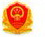中国航空 运输协会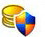企业营业 执照认证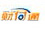支付宝 认证商家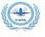民航客运 一类代理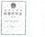旅行社资质

 航空公司 四川航空公司 上海航空公司 海南航空公司 中国国际航空公司 厦门航空公司 中国东方航空公司 山东航空公司 深圳航空公司 中国联合航空有限公司 云南祥鹏航空公司 中国春秋航空公司 中国南方航空公司 奥凯航空有限公司 鹰联航空公司 华夏航空公司 吉祥航空公司 西部航空公司 大新华航空公司 河南航空有限公司 东北航空公司 重庆航空公司